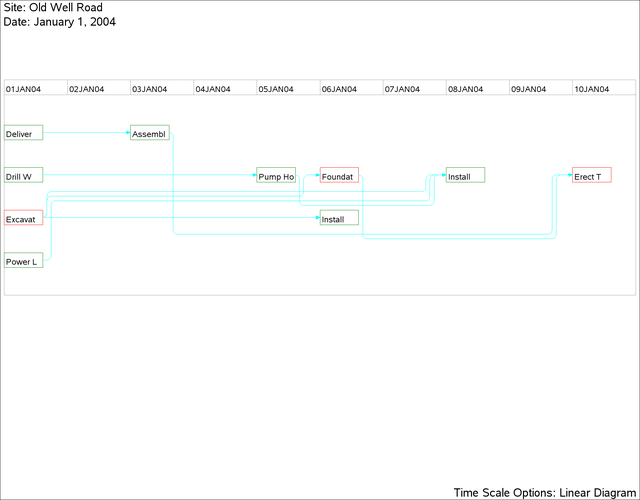Example 9.10 Further Time-Scale Options

In this example, the construction project described in Example 9.7 is used to illustrate some more time-scale options. First, the REFBREAK option indicates breaks in the time axis by drawing a zigzag line down the diagram before each tick mark corresponding to a break. The CREFBRK= and LREFBRK= options control the color and line style for these lines. The network diagram is shown in Output 9.10.1.

```
title j=l h=1.5 ' Site: Old Well Road';
title2 j=l h=1.5 ' Date: January 1, 2004';
footnote j=r h=1.5 'Time Scale Options: Reference Breaks ';

proc netdraw data=sched graphics;
dur = duration
succ = (succesr1-succesr3)
dp compress separatearcs
font=swiss htext=2
timescale refbreak lrefbrk = 33
carcs=cyan crefbrk = blue;
run;
```

Output 9.10.1: Time-Scaled Network: Reference BreaksNext, PROC NETDRAW is invoked with the LINEAR option so that there is no break in the time axis. The BOXWIDTH= option limits the size of each node. The diagram is drawn in Output 9.10.2.

```
title j=l h=1.5 ' Site: Old Well Road';
title2 j=l h=1.5 ' Date: January 1, 2004';
footnote j=r h=1.5 'Time Scale Options: Linear Diagram ';

proc netdraw data=sched graphics;
dur = duration
succ = (succesr1-succesr3)
dp
pcompress
novcenter
vmargin = 10
separatearcs
htext=2
carcs=cyan